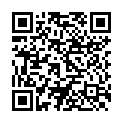# North Coast Synthesis Ltd.

## G♭+2+11+♯2Choose audio sample set:
MSK 007 Leapfrog VCF (patch; see in online store)
MSK 009 Coiler VCF (patch; see in online store)

Equivalent chord symbols: F♯+2+4+♯2, F♯+4+9+♯2, G♭+2+4+♯2, G♭+4+9+♯2, F♯+2+11+♯2.

Notes in this chord: G♭, A♭, A, B♭, D♭, C♭. Integer notation: {1, 6, 8, 9, 10, 11}.

Nearby chords (one less note): G♭+2+4, G♭+2+♯2, G♭+4+♯2, G♭m+2+4, G♭4+2+♯2, A♭m4+2+♯1.

Nearby chords (one more note): G♭11+♯2, A♭m11+♯1, E13-1+♯4, G♭M11+♯2, G♭+2+4+♯1+♯2, G♭+2+4+♯2+♯4.

Parallel chords (same structure, different root): C+2+11+♯2, D+2+11+♯2, E+2+11+♯2, F+2+11+♯2, G+2+11+♯2, A+2+11+♯2, B+2+11+♯2, C♭+2+11+♯2, D♭+2+11+♯2, E♭+2+11+♯2, F♭+2+11+♯2, A♭+2+11+♯2, B♭+2+11+♯2, C♯+2+11+♯2, D♯+2+11+♯2, E♯+2+11+♯2, F♯+2+11+♯2, G♯+2+11+♯2, A♯+2+11+♯2, B♯+2+11+♯2.

Experimental fretting charts for guitar standard EADGBE tuning (change tuning or instrument):